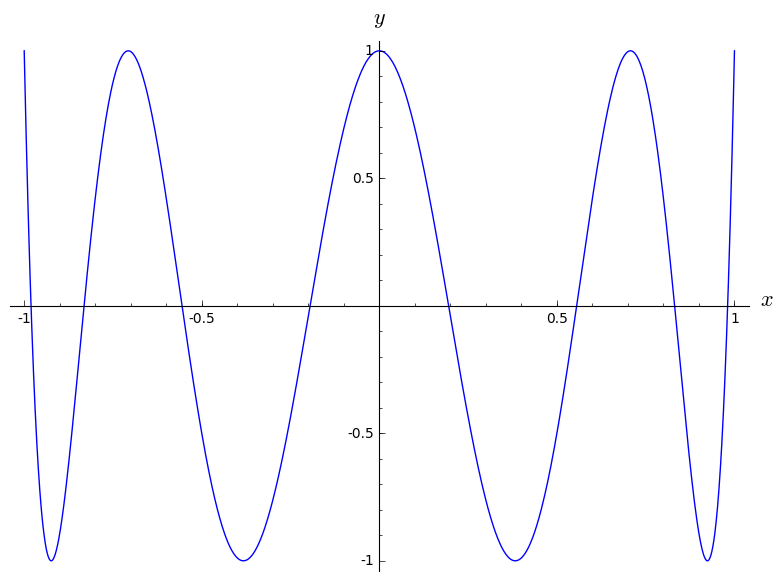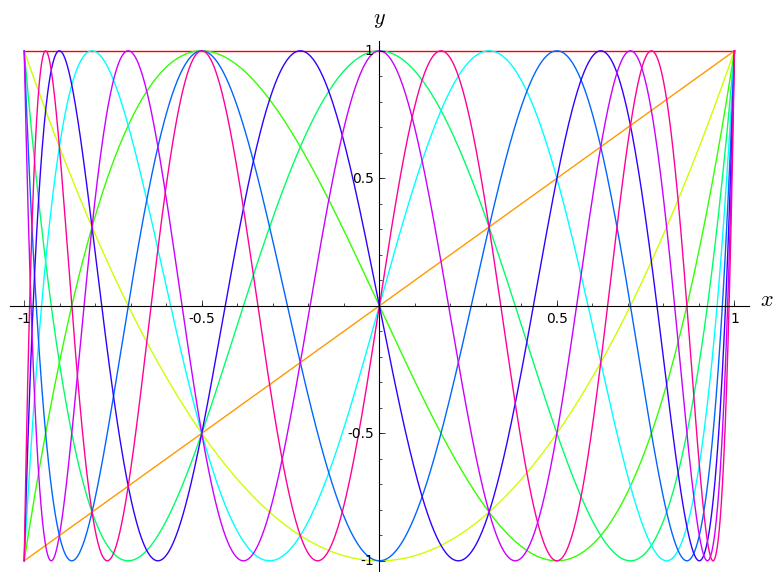# A quick tour of SageMath¶

This worksheet illustrates a few elementary features of SageMath.

First we set up the display to have nice LaTeX output:

In :
%display latex


SageMath knows about $\pi$, $e$ and $i$ (well, it's a mathematical software, isn't it ?):

In :
e^(i*pi) + 1

Out:

SageMath can compute numerical values with an arbitrary number of digits:

In :
n(pi, digits=1000)

Out:

Another interesting computation regards the Hermite-Ramanujan constant:

In :
a = exp(pi*sqrt(163))
a

Out:

Actually, this number is very close to an integer, as announced by Charles Hermite in 1859 (probably without using SageMath...):

In :
n(a, digits=50)

Out:

That's clear if we turn off scientific notation:

In :
n(a, digits=50).str(no_sci=2)

Out:

Beside numerical computations, SageMath can perform symbolic ones, such as taking a derivative:

In :
f = diff(sin(x^2),x) ; f

Out:

By default, SageMath displays all results, such as the one above, in LaTeX format. The explicit LaTeX code can be shown:

In :
print(latex(f))

2 \, x \cos\left(x^{2}\right)


SageMath can also compute integrals:

In :
integrate(x^5/(x^3-2*x+1), x, hold=True)

Out:
In :
integrate(x^5/(x^3-2*x+1), x)

Out:
In :
integrate(exp(-x^2), x, -oo, +oo)

Out:

As in many computer algebra systems, the documentation is provided after a question mark:

In :
diff?


What singularizes SageMath is the double question mark: it returns the Python source code ! Indeed SageMath is a free software and allows for an easy access to the source code:

In :
diff??


Other examples of computations: Taylor series:

In :
exp(x).series(x==0, 8)

Out:

and Riemann's zeta function $\zeta(s)$ for $s=2$ and $s=3$ (Apéry's constant):

In :
var('n') # declaring n as a symbolic variable
sum(1/n^2, n, 1, +oo)

Out:
In :
sum(1/n^3, n, 1, +oo)

Out:

As any decent mathematical software, SageMath has some plotting capabilities:

In :
plot(chebyshev_T(8,x),(x,-1,1), axes_labels=['$x$', '$y$'])

Out:To illustrate the advantage of being built atop of Python, let us write a loop to draw the first ten Chebyshev polynomials. We simply use standard Python syntax (no need to learn some specific script language!):

In :
g = Graphics()
for i in range(10):
g += plot(chebyshev_T(i,x), (x,-1,1), color=hue(i/10))
show(g, axes_labels=['$x$', '$y$'])Another example of Python syntax in SageMath: displaying Pascal's triangle with only two instruction lines:

In :
for n in range(10):
print([binomial(n,p) for p in range(n+1)])


[1, 1]
[1, 2, 1]
[1, 3, 3, 1]
[1, 4, 6, 4, 1]
[1, 5, 10, 10, 5, 1]
[1, 6, 15, 20, 15, 6, 1]
[1, 7, 21, 35, 35, 21, 7, 1]
[1, 8, 28, 56, 70, 56, 28, 8, 1]
[1, 9, 36, 84, 126, 126, 84, 36, 9, 1]


SageMath's graphic capabilities extend to 3-D:

In :
dodecahedron()

Out:
In :
x, y = var('x y')
show(plot3d(sin(x*y), (x,-pi,pi), (y,-pi,pi), color='green'), viewer='threejs')


Among other things, SageMath is quite developed in number theory. Let us just show primality tests of two Mersenne numbers:

In :
n = 2^31-1 ; n

Out:
In :
n.is_prime()

Out:
In :
n = 2^61-1 ; n

Out:
In :
n.is_prime()

Out:

SageMath is also well developed in group theory. A very small overview of its capabilities:

In :
S4 = SymmetricGroup(4) ; print S4

Symmetric group of order 4! as a permutation group

In :
S4.list()

Out:
In :
S4.is_abelian()

Out:
In :
g = S4([2,1,4,3]) ; g

Out:
In :
g.domain()

Out:
In :
g.sign()

Out:
In :
h = S4([3,1,2,4]) ; h

Out:
In :
s = g*h ; s

Out:
In :
s.inverse()

Out: